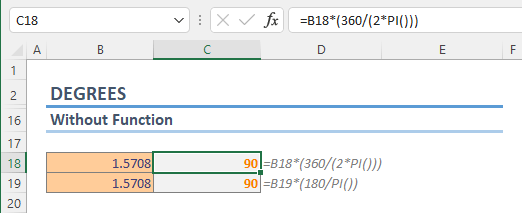In Excel, The DEGREES function is a Math & Trigonometry function that converts radians into degrees. In this guide, we’re going to show you how to use the DEGREES function and go over some tips and error handling methods.

• All versions

DEGREES(angle)

## Arguments

 angle A radian value that you want to convert.

## Examples

The radian is a unit of measurement of angles. One radian is defined as the angle subtended from the center of a circle which intercepts an arc equal in length to the radius of the circle.

The math behind calculation is simple:Which can be simplified into:You can use PI function to return π value.

All you need to do is to supply the angle degreed you want to convert.

=DEGREES(3.14159265358979)

=DEGREES(PI())